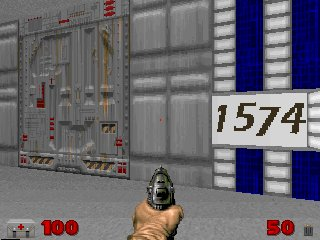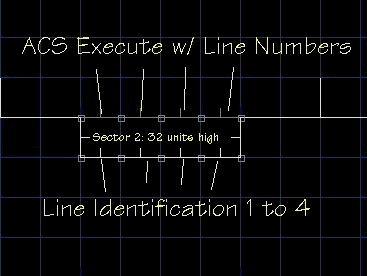# Knowledge Base - Combo Lock

## Combo LockFigure 1: What's the Combination?

ZDoom offers the level designer a full toolbox to allow player interaction with the Doom environment. In Figure 1, the player must hit the right combination to open the door. When the player stands in front of a number and presses the uses key, the number displayed (in really bad graphics :), changes. When the right combination of numbers are displayed, the door opens. The principles in this tutorial can be used in a wide range of applications.Figure 2: Map Detail

Figure 2 illustrates the actual lock in the example map, comblock.wad. Sector 2 is set to 32 units high and has the facing lines split into four segments. Each of the one-sided linedef segments have a graphic 16x32 that displays a number from 0 to 9. The one sided linedef segments each have a different line identification so that the graphics can be set individually. The line setup is illustrated in Figure 3.Figure 3: Line Identification Special

The circled parameter is the line identification number. The lines are numbered 1 to 4. In order to change the texture from a script, the line has to be identified to ACS and the identification special allows ACS to operate on the correct line.

The double sided linedefs also have a special associated with them. In this case, each line when it is used (i.e., when the player presses the use key), the line will call a script with the associated line identification number.Figure 4: Execute Script

Figure 4 illustrates the setup for the double sided linedefs. When the player presses the uses key, script 2 is executed on the current map. The first two parameters in the dialog are the script number and map id as shown in other tutorials. The third parameter (circled parameter), is the line identification number set on the single-sided linedef. The script requires this number so it will know which texture to change.

The setup here may be a bit confusing at first glance. What I have done is paired a double sided linedef with a single sided linedef. The single sided linedef is used to display the graphic while the double sided is used to allow the player to interact with the lock. Pressing the use key allows the player to change the number on the current segment. When the number gets to 9, it wraps around to 0.

The heart of this construct is the ACS script. Here is the script in its entirety.

```#include "zcommon.acs"

int digit1;
int digit2;
int digit3;
int digit4;

script 1 OPEN
{
int tcnt;
int rantex;

tcnt = 1;
// Set the digits on the lock.
while (tcnt < 5)
{
// Choose a random digit.
rantex = random(const:0,9);

// Save the value for later.
switch (tcnt)
{
case 1:
digit1 = rantex;
break;
case 2:
digit2 = rantex;
break;
case 3:
digit3 = rantex;
break;
case 4:
digit4 = rantex;
break;
}

// Set the texture on the digit.
ACS_Execute (100, 0, tcnt, rantex, 0);

// Get the next line.
tcnt++;

Delay(35);
}

}

// This script increments the current digit.
script 2 (int line)
{
int tex;

switch (line)
{
case 1:
digit1++;
if (digit1 > 9)
digit1 = 0;
tex = digit1;
break;
case 2:
digit2++;
if (digit2 > 9)
digit2 = 0;
tex = digit2;
break;
case 3:
digit3++;
if (digit3 > 9)
digit3 = 0;
tex = digit3;
break;
case 4:
digit4++;
if (digit4 > 9)
digit4 = 0;
tex = digit4;
break;
}

// Set the texture on the digit.
ACS_Execute (100, 0, line, tex, 0);

// See if the combo hit.
if (digit1 == 6 && digit2 == 6 && digit3 == 6 && digit4 == 6)
Door_Open (1, 64);
}

// Sets the line texture. Acts as a subroutine.
script 100 (int line, int num)
{

switch (num)
{
case 0:
SetLineTexture(line, SIDE_FRONT, TEXTURE_MIDDLE, "0");
break;
case 1:
SetLineTexture(line, SIDE_FRONT, TEXTURE_MIDDLE, "1");
break;
case 2:
SetLineTexture(line, SIDE_FRONT, TEXTURE_MIDDLE, "2");
break;
case 3:
SetLineTexture(line, SIDE_FRONT, TEXTURE_MIDDLE, "3");
break;
case 4:
SetLineTexture(line, SIDE_FRONT, TEXTURE_MIDDLE, "4");
break;
case 5:
SetLineTexture(line, SIDE_FRONT, TEXTURE_MIDDLE, "5");
break;
case 6:
SetLineTexture(line, SIDE_FRONT, TEXTURE_MIDDLE, "6");
break;
case 7:
SetLineTexture(line, SIDE_FRONT, TEXTURE_MIDDLE, "7");
break;
case 8:
SetLineTexture(line, SIDE_FRONT, TEXTURE_MIDDLE, "8");
break;
case 9:
SetLineTexture(line, SIDE_FRONT, TEXTURE_MIDDLE, "9");
break;
}
}
```

The first thing to do is create some variables for the different digits.

```int digit1;
int digit2;
int digit3;
int digit4;
```

These variables are used in two ways: to display the proper graphic and to hold the actual number the graphic represents. When the player changes the combination, the script will check these variables to see if the right combination has been entered.

Script 1 is declared an OPEN script which means it will execute when the map is loaded. Script 1 initializes the combination lock. It loops through each line number and sets the texture on the single sided linedef segment shown in Figure 2, and loads that value in the digit variable for use later. tcnt and randtex are simply working variables that will be used in the script.

The while loop starts at 1 and executes the statement within the { and } until tcnt reaches 5 where the script will continue. Inside the braces, a random number is generated and saved in randtex. The script then executes a switch statement to save the value in the proper digit variable.

The switch statement is an easier to use if-then-else if-else construct. The value of tcnt determines which case is executed. On the first pass, tcnt is 1, so case 1 is executed. The variable digit1 is set to the value of randtex and then a break is executed. Break simply means get out of the switch statement. (The switch is the C version of the VB SELECT CASE.)

After the switch statement, (this is still part of the while loop), the script executes another script, script 100, via the ACS Execute command.

Script 100 here is a separate script because it is being used as a subroutine (or method call if you prefer). It is actually called here in script 1 and in script 2. Instead of duplicating the code in both scripts, I simply placed this code in a third script to be called by any other script.

Script 1 then simply delays for a tic, and continues on in the while loop. It will execute each of these commands for each line segment.

Script 2 is called when the player uses a particular line segment. It takes one parameter, the line identification number. Once again a switch statement is called to determine which line to change.

```            digit1++;
if (digit1 > 9)
digit1 = 0;
tex = digit1;
```

In the code snippet, line one has been used by the player. The digit variable is incremented by one. Then the script checks to see if the number is out of range, or greater than 9. If it is, the variable is set to 0. This makes the numbers appear to wrap around when the player uses them. Another working variable is then set to be used in another script 100 subroutine call, via the ACS Execute statement.

The last thing we need to do is check the lock for the proper combination. The if statement looks to see if all the variables are 6 (of course :) and if they are, the Door Open special is executed.

The visual effects of the combo lock come from script 100. The parameters for the script are the line number and the graphic number to display. Inside the appropriate case, the SetLineTexture special is called to change the texture on the single sided line segment.

SetLineTexture (line, side, position, texture)

• line: The line identification number.
• side: The linedef side. This is either SIDE_FRONT, or SIDE_BACK. This corresponds to what is termed in some editors as the "right" side. The right and front side are the same. It is the side that has the little line sticking out from it in Figure 2.
• position: This is the texture to change and may be TEXTURE_TOP, TEXTURE_MIDDLE or TEXTURE_BOTTOM.
• texture: This is the texture name as a string value ("BFALL1").

Note: To use the readable constants, you must have the #include "zcommon.acs" statement in the script. It is best to place this at the top of the script.

In script 100, the "used" line texture is set to the proper digit graphic depending on the value of the digit variable. As the player uses the line, the numbers will change for that line segment.

This ugly little lock offers a another level of interactivity for the level designer, but the principles used here can be used in other applications as well and illustrate that scripts can interact with both the world and each other.

### Sources

ZDoom reference by Marisa Heit.
3D Game Alchemy by Steve Benner, et al. Copyright © 1996 By Sams Publishing

Back